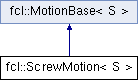FCL  0.6.0 Flexible Collision Library
fcl::ScrewMotion< S > Class Template Reference
Inheritance diagram for fcl::ScrewMotion< S >:## Public Member Functions

ScrewMotion ()
Default transformations are all identities.

ScrewMotion (const Matrix3< S > &R1, const Vector3< S > &T1, const Matrix3< S > &R2, const Vector3< S > &T2)
Construct motion from the initial rotation/translation and goal rotation/translation.

ScrewMotion (const Transform3< S > &tf1_, const Transform3< S > &tf2_)
Construct motion from the initial transform and goal transform.

bool integrate (double dt) const
Integrate the motion from 0 to dt We compute the current transformation from zero point instead of from last integrate time, for precision.

computeMotionBound (const BVMotionBoundVisitor< S > &mb_visitor) const
Compute the motion bound for a bounding volume along a given direction n, which is defined in the visitor.

computeMotionBound (const TriangleMotionBoundVisitor< S > &mb_visitor) const
Compute the motion bound for a triangle along a given direction n, which is defined in the visitor.

void getCurrentTransform (Transform3< S > &tf_) const
Get the rotation and translation in current step.

void getTaylorModel (TMatrix3< S > &tm, TVector3< S > &tv) const

getLinearVelocity () const

getAngularVelocity () const

const Vector3< S > & getAxis () const

const Vector3< S > & getAxisOrigin () constPublic Member Functions inherited from fcl::MotionBase< S >
virtual bool integrate (S dt) const =0
Integrate the motion from 0 to dt.

void getCurrentTransform (Matrix3< S > &R, Vector3< S > &T) const
Get the rotation and translation in current step.

void getCurrentTransform (Quaternion< S > &Q, Vector3< S > &T) const

void getCurrentRotation (Matrix3< S > &R) const

void getCurrentRotation (Quaternion< S > &Q) const

void getCurrentTranslation (Vector3< S > &T) const

const std::shared_ptr< TimeInterval< S > > & getTimeInterval () const

## Protected Member Functions

void computeScrewParameter ()

Quaternion< S > deltaRotation (S dt) const

Quaternion< S > absoluteRotation (S dt) const

## Protected Attributes

Transform3< S > tf1
The transformation at time 0.

Transform3< S > tf2
The transformation at time 1.

Transform3< S > tf
The transformation at current time t.

Vector3< S > axis
screw axis

Vector3< S > p
A point on the axis.

linear_vel
linear velocity along the axis

angular_vel
angular velocityProtected Attributes inherited from fcl::MotionBase< S >
std::shared_ptr< TimeInterval< S > > time_interval_

The documentation for this class was generated from the following files: